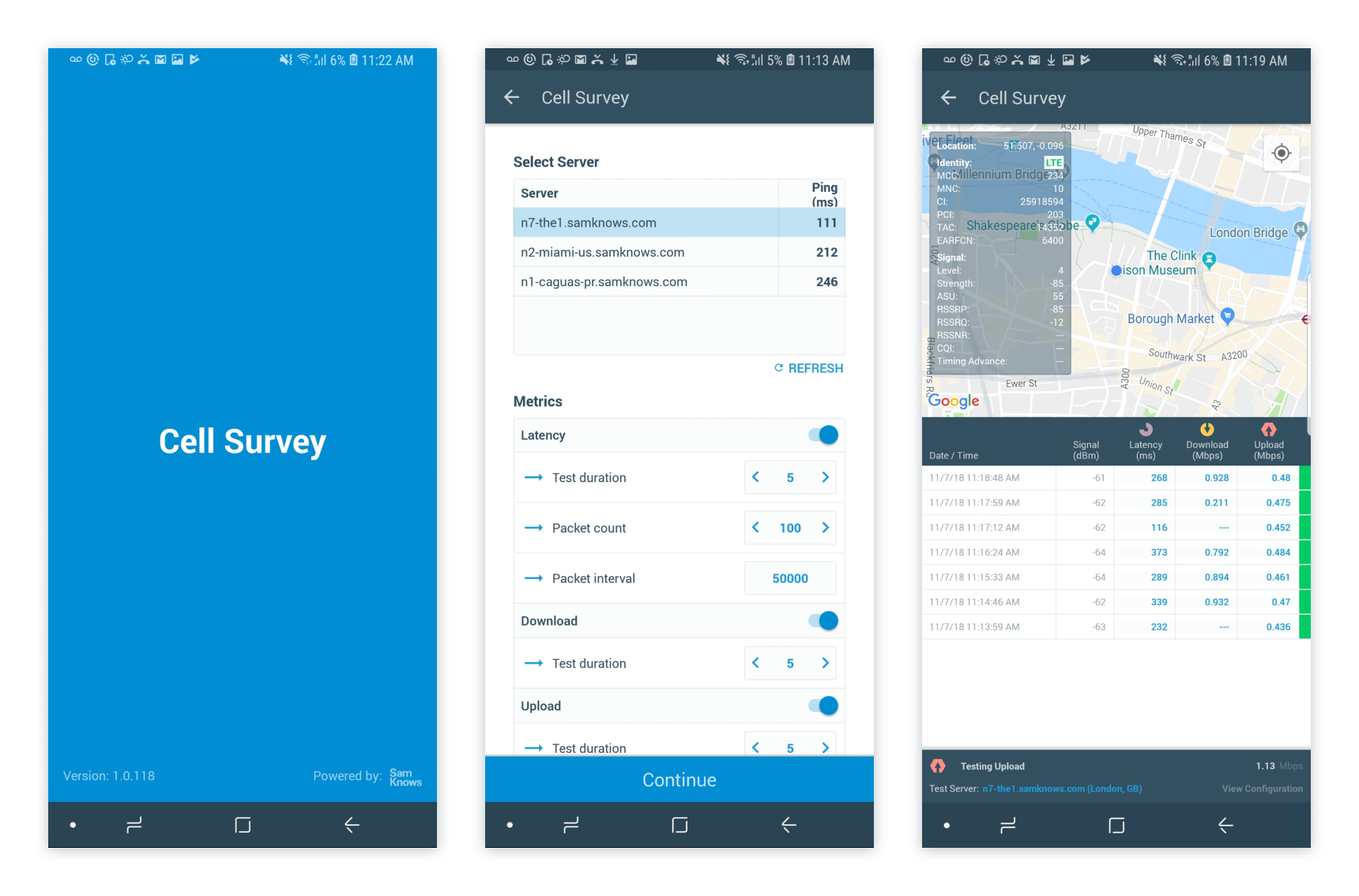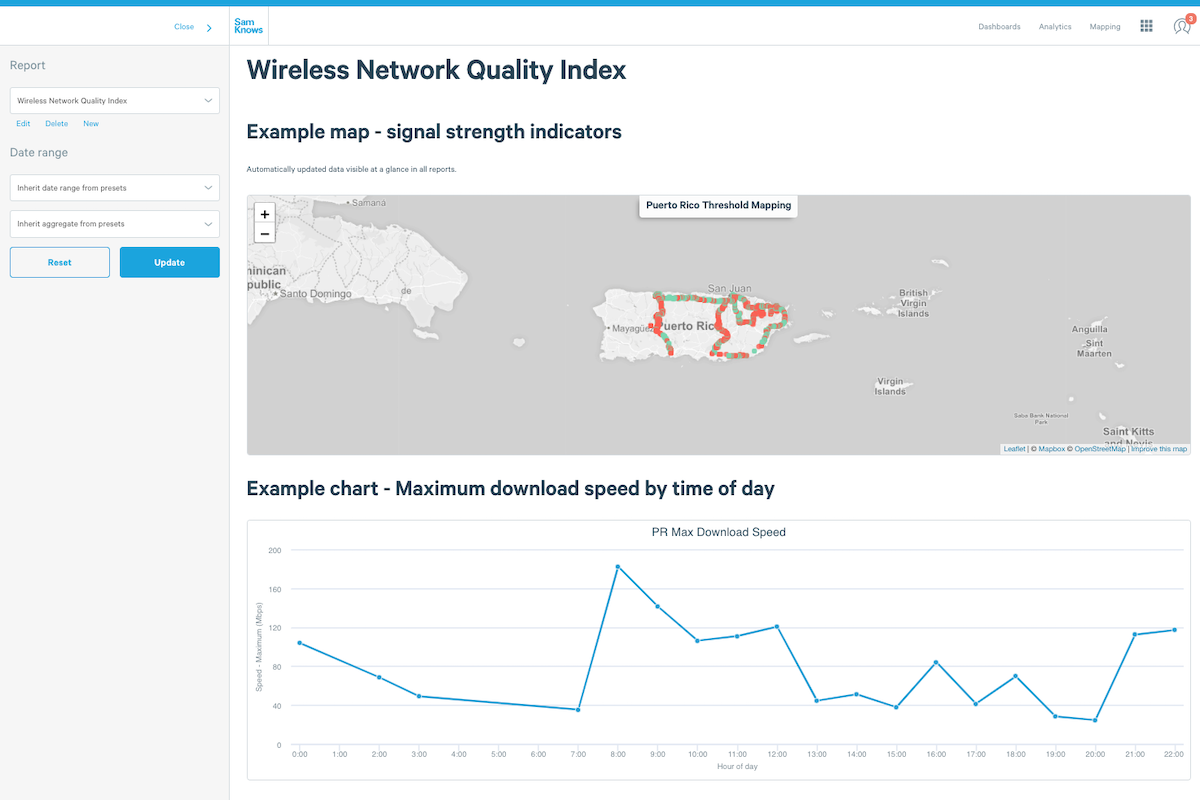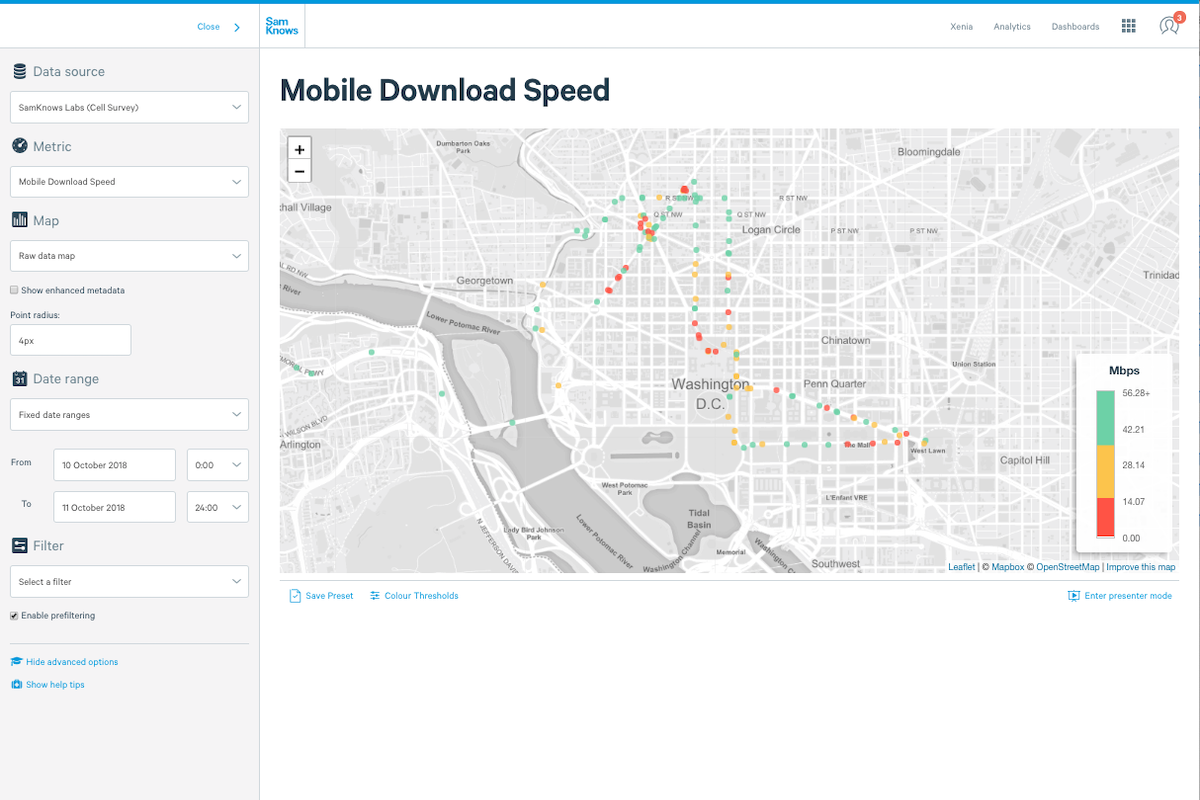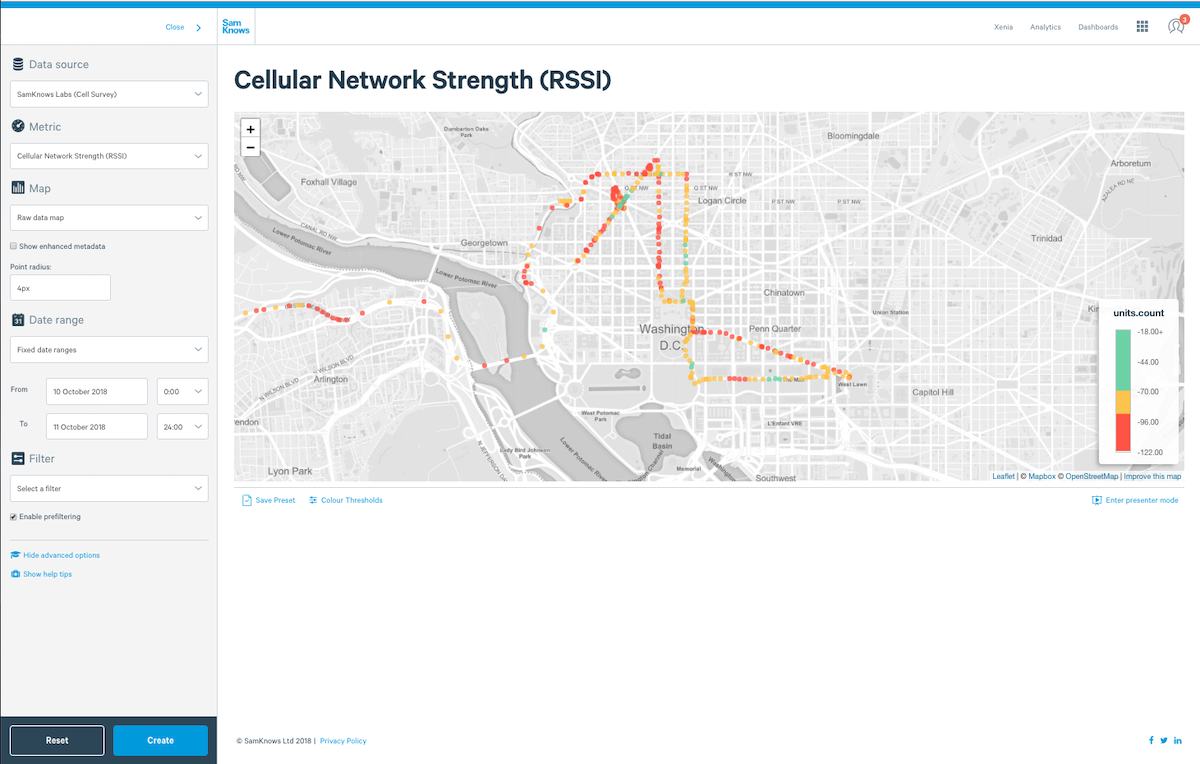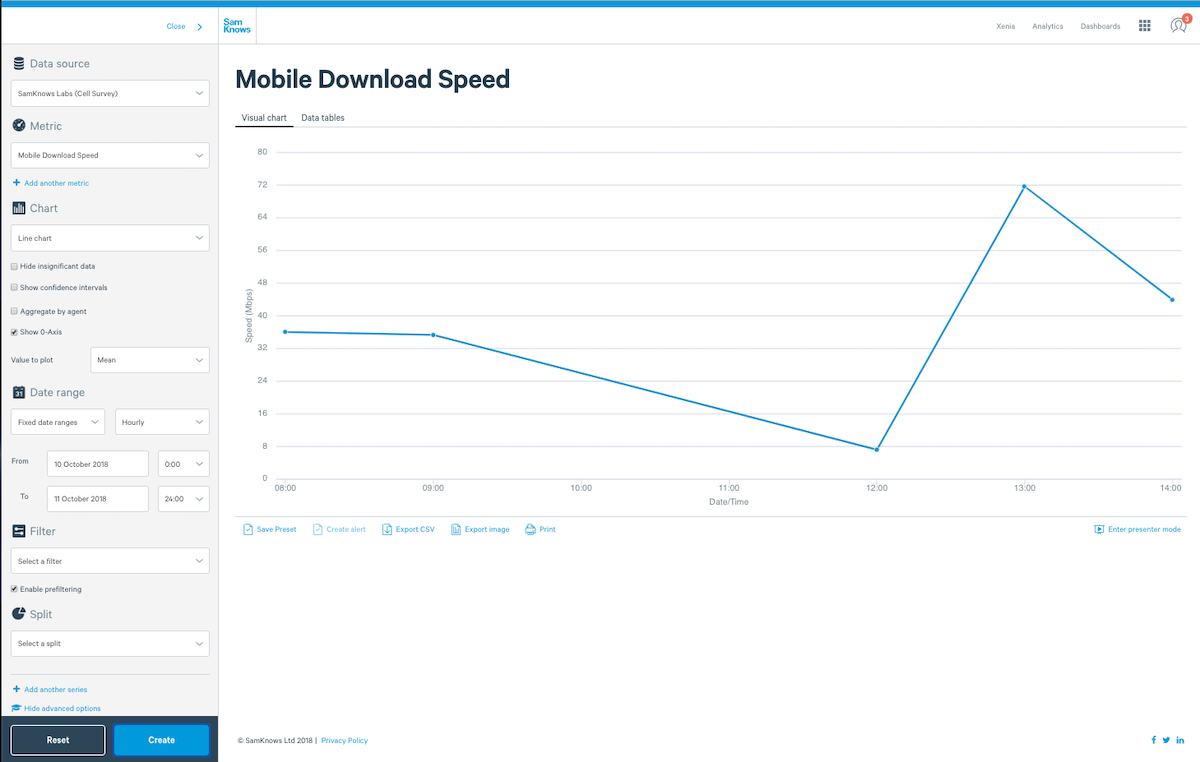# Regulatory compliance.

The SamKnows products and services are often used by ISPs to meet regulation. Sometimes we create tailor-made products to solve particularly complex regulatory or advertising requirements.

## Ofcom Voluntary Code of Practice

The Ofcom Voluntary Code of Practice is very important within the UK Broadband market and as an ISP, whether you are a signatory or not, you are probably affected by it and looking to measure your data. This product is based on the metrics set out in Annex 5 of the Voluntary Codes of Practice on Better Broadband Speeds to help you determine what your Normally Available, Maximum and Minimum speeds are and monitor them for any change. It fully supports xDSL and FTTP/Cable metric types and has Business and Consumer broadband variants. The metrics are fully integrated into SamKnows One allowing you to utilise the full power of SamKnows One analytics to use these metrics in alerting, dashboards, presets, data API exports or reports.

We automatically perform actions such as prefiltering down to results that ran for the correct duration and with the appropriate thread count to meet VCOP guidelines on all metrics and we also perform aggregations of agent results before aggregating over the entire dataset with the Advanced Analytics product.

The following metrics are provided to FTTP/Cable Providers:

We filter down to tests during quiet hour or hours then take the mean average of a measurement agents' results in the time bucket specified (e.g. a week), then we calculate the 80th percentile over those results.

### VCOP Maximum Upload (FTTP / Cable)

We filter down to tests during quiet hour or hours then take the mean average of a measurement agents' results in the time bucket specified (e.g. a week), then we calculate the 80th percentile over those results.

We filter down to tests during peak hours (8-10pm) then take a mean average of a measurement agents' results in the time bucket (e.g. a week) then we calculate the 80th percentile over those results.

### VCOP Residential Normally Available Upper Upload (FTTP / Cable)

We filter down to tests during peak hours (8-10pm) then take a mean average of a measurement agents' results in the time bucket (e.g. a week) then we calculate the 80th percentile over those results.

We filter down to tests during peak hours (8-10pm) then take a mean average of a measurement agents' results in the time bucket (e.g. a week) then we calculate the 20th percentile over those results.

### VCOP Residential Normally Available Lower Upload (FTTP / Cable)

We filter down to tests during peak hours (8-10pm) then take a mean average of a measurement agents' results in the time bucket (e.g. a week) then we calculate the 20th percentile over those results.

### VCOP National Congestion Residential Peak Coefficient (xDSL)

We filter down to tests during peak hours (8-10pm) then take a mean average of a measurement agents' results in the time bucket (e.g. a week) then we calculate a delta between that number and the measurement agents' sync-speed (defined in a metadata field) then return the average of these deltas.

### VCOP National Congestion Off-Peak Coefficient (xDSL)

We filter down to tests during quiet hour or hours (currently 2am-6am for Virgin) then take a mean average of a measurement agents' results in the time bucket (e.g. a week) then we calculate a delta between that number and the measurement agents' sync-speed (defined in a metadata field) then return the average of these deltas.

We apply that week's pre-derived national off-peak coefficient for the agent's current [at the time of the test] package to a measurement agents' results, then derive the mean average of those derived agent results in the time bucket specified (e.g. a month), then we calculate the 80th percentile over those results.

We apply that week's pre-derived national off-peak coefficient for the agent's current [at the time of the test] package to a measurement agents' results, then derive the mean average of those derived agent results in the time bucket specified (e.g. a month), then we calculate the 80th percentile over those results.

We apply that week's pre-derived national residential peak coefficient for the agent's current [at the time of the test] package to a measurement agents' results, then derive the mean average of those derived agent results in the time bucket specified (e.g. a month), then we calculate the 80th percentile over those results.

### VCOP Residential Normally Available Upper Upload (xDSL)

We apply that week's pre-derived national residential peak coefficient for the agent's current [at the time of the test] package to a measurement agents' results, then derive the mean average of those derived agent results in the time bucket specified (e.g. a month), then we calculate the 80th percentile over those results.

We apply that week's pre-derived national residential peak coefficient for the agent's current [at the time of the test] package to a measurement agents' results, then derive the mean average of those derived agent results in the time bucket specified (e.g. a month), then we calculate the 20th percentile over those results.

### VCOP Residential Normally Available Lower Upload (xDSL)

We apply that week's pre-derived national residential peak coefficient for the agent's current [at the time of the test] package to a measurement agents' results, then derive the mean average of those derived agent results in the time bucket specified (e.g. a month), then we calculate the 20th percentile over those results.

We apply that week's pre-derived national residential peak coefficient for the agent's current [at the time of the test] package to a measurement agents' results, then derive the mean average of those derived agent results in the time bucket specified (e.g. a month), then we calculate the 10th percentile over those results.

### VCOP Residential Minimum Upload (xDSL)

We apply that week's pre-derived national residential peak coefficient for the agent's current [at the time of the test] package to a measurement agents' results, then derive the mean average of those derived agent results in the time bucket specified (e.g. a month), then we calculate the 10th percentile over those results.

We filter down to tests during peak hours (12pm-2pm) then take a mean average of a measurement agents' results in the time bucket (e.g. a week) then we calculate the 20th percentile over those results.

We filter down to tests during peak hours (12pm-2pm) then take a mean average of a measurement agents' results in the time bucket (e.g. a week) then we calculate the 80th percentile over those results.

We filter down to tests during peak hours (12pm-2pm) then take a mean average of a measurement agents' results in the time bucket (e.g. a week) then we calculate the 80th percentile over those results.

We filter down to tests during peak hours (12pm-2pm) then take a mean average of a measurement agents' results in the time bucket (e.g. a week) then we calculate the 20th percentile over those results.

The following metrics are provided to xDSL Providers:

We apply that week's pre-derived national residential off-peak coefficient for the agent's current [at the time of the test] package to a measurement agents' results, then derive the mean average of those derived agent results in the time bucket specified (e.g. a month), then we calculate the 80th percentile over those results.

### VCOP National Congestion Business Peak Coefficient (xDSL)

We filter down to tests during peak hours (12pm-2pm) then take a mean average of a measurement agents' results in the time bucket (e.g. a week) then we calculate a delta between that number and the measurement agents' sync-speed (defined in a metadata field) then return the average of these deltas.

We apply that week's pre-derived national residential off-peak coefficient for the agent's current [at the time of the test] package to a measurement agents' results, then derive the mean average of those derived agent results in the time bucket specified (e.g. a month), then we calculate the 80th percentile over those results.

We apply that week's pre-derived national residential off-peak coefficient for the agent's current [at the time of the test] package to a measurement agents' results, then derive the mean average of those derived agent results in the time bucket specified (e.g. a month), then we calculate the 20th percentile over those results.

We apply that week's pre-derived national residential off-peak coefficient for the agent's current [at the time of the test] package to a measurement agents' results, then derive the mean average of those derived agent results in the time bucket specified (e.g. a month), then we calculate the 20th percentile over those results.

We apply that week's pre-derived national residential off-peak coefficient for the agent's current [at the time of the test] package to a measurement agents' results, then derive the mean average of those derived agent results in the time bucket specified (e.g. a month), then we calculate the 10th percentile over those results.

We apply that week's pre-derived national residential off-peak coefficient for the agent's current [at the time of the test] package to a measurement agents' results, then derive the mean average of those derived agent results in the time bucket specified (e.g. a month), then we calculate the 10th percentile over those results.

## ACCC Typical Busy Period Speeds

The Australian Competition & Consumer Commission have published industry guidance on the advertising speeds of broadband. This introduces a standardised metric that characterises the typical busy period speeds for each broadband plan that an RSP offers ('RSP' is the common term for a retail internet service provider in Australia).

SamKnows has been extended to support the ACCC typical speed metrics natively. These allow you to visualise the typical busy period speeds, which are presented separately for download and upload speed. The metrics are fully integrated into SamKnows One, meaning that they can be used in data analysis, alerting, dashboards, presets, data API exports and reports.

### Metrics

We offer four metrics that perform the above calculations:

2. Typical Busy Period - Upload Speed

### Calculations

We calculate the metric in the same way we do for both the ACCC reports and in SamKnows One. The approach is described as follows.

1. We calculate the metric in the same way we do for both the ACCC reports and in SamKnows One. The approach is described as follows.
2. Average across all Agents, by day and hour. This should result in 56 records in total across a two week period.
3. Pick the weeks + 1th lowest result. Across a two week period, this would mean picking the 3rd lowest result. This is the typical evening speed for the plan.

The result can be presented in megabits per second, or alternatively as a percentage of the plan's advertised speeds.

## FCC Mobility Fund II

The FCC Mobility Fund II provides money for qualifying operators to invest in providing mobile broadband to unserved areas of the United States of America. In order to qualify it must be proven that the current available service is less than 5 megabits per second. SamKnows has built a kit, specifically for this purpose, which is now being used by the FCC.

This can be used by:

1. Challengers to prove coverage is below the 5 megabits per second minimum benchmark and an area is eligible for funding
2. By incumbent carriers to prove the accuracy of existing coverage maps
3. By federal and state regulators to qualify statements made by industry relating to coverage in particular areas
4. By federal and state regulators to monitor the impact on investment in areas where coverage is received.

The Wireless Network Quality kit allows for the following active measurements to run.

### Active measurements

These tests measure the download HTTP throughput in bits per second. The test will run for a fixed duration (in addition to a TCP warmup period). This time is configurable at runtime.

The duration default is 5 seconds, maximum is 30 seconds and minimum is 5 seconds.

These tests measure the upload HTTP throughput in bits per second. The test will run for a fixed duration (in addition to a TCP warmup period). This time is configurable at runtime.

The duration default is 5 seconds, maximum is 30 seconds and minimum is 5 seconds.

#### Latency / packet loss / jitter

This is a single test that provides three separate metrics upon completion: latency, jitter and packet loss. Only latency is shown in-app but all three metrics are submitted and visible in SamKnows One. You can configure the packet count, packet interval and test duration at runtime.

The duration default is 5 seconds, maximum is 30 seconds and minimum is 5 seconds. The packet count default is 200, maximum is 500, and minimum is 100.

It also captures supporting evidence in the form of environmental data, which includes key metrics like:

• Handset type
Capturing the handset model ensures results are demonstrably compliant with the FCC’s list of accepted handsets for testing.
• Failed test capture
Demonstrating areas of low- or no-coverage by capturing tests that were not able to complete due to poor network proves eligibility across tested areas.
• Cell tower ID
Understanding which cell tower was providing network for each test can indicate cell loading factor and demonstrate eligibility